# PID Control Tutorial

### Overview

PID is an acronym for proportional band, integral and derivative. This control action allows a measurement (process variable) to be controlled at a desired set point by continuously adjusting a control output. These control parameters act on the error or deviation between set point and process variable.

P: Proportional Band in %
I: Integral Time in sec/repeat
D: Derivative Time in seconds

### Proportional Control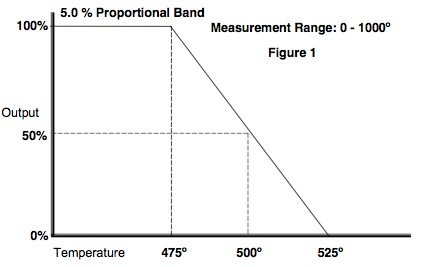With proportional band, the controller output changes in "proportion" to the error between process variable and set point. The amplitude of the change is adjustable from 1% to 999.9%.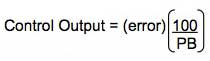Refer to Figure 1 below. This example of a temperature controller shows a proportional band setting of 5%.

Set point = 500°
Measurement range = 0-1000°
5% PB = 5% of 1000° = 50°
100% output at 475° (2.5% of 1000°)
0% output at 525° (2.5% of 1000°)

If the process variable equals the set point (500°), there is a 50% output. As the temperature decreases, the proportional band increases the output linearly toward 100% as the temperature falls toward 475°. The output decreases below 50% as the temperature rises toward 525°.

In this example, a small change in temperature provides a large change in output. If the setting is too small for the process dynamics, oscillations will occur and will not settle at set point. A large PB setting makes the controller act sluggish and will not respond adequately to upsets. Since proportional control does not incorporate the time that the error has existed, there will always be an offset from set point.

Typically, flow or pressure controllers have a much larger proportional setting due to a possible narrower measurement range and fast process reaction to a change in the control output. For example, a flow controller may have an input range of 0 to 60gpm and a set point of 30gpm.

Measurement range = 60gpm
Set point = 30gpm
100% PB = 100% of 60 = 60gpm
100% output at 0gpm
0% output at 60gpm

### Proportional + Manual Reset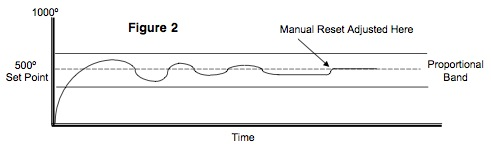To eliminate the inherent offset observed with proportional control, a manual reset function can be used. Virtually no process requires precisely 50% output to maintain the process variable at the set point. An offset will be present. Manual reset allows the user to bias the output accordingly to compensate for the steady state offset using P only. Refer to Figure 2 below.

### Proportional + Automatic Reset (Integral)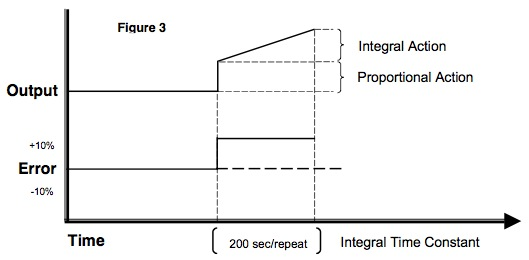Automatic reset or integral action corrects for any offset between set point and process variable automatically by shifting the proportional band over a pre-defined time. The integral time repeats the proportional action over the time set. Integral redefines the output requirements at the set point until the process variable and set point are equal. Integral engineering units vary by controller manufacturer. Some use repeats/minute (reset rate), minutes/repeat or seconds/repeat. In Figure 3 below, seconds/repeat is used. The integral term is added as follows: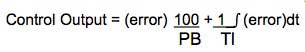The smaller the integral number, the proportional action will be repeated more often. If integral is too small, the process variable will oscillate through set point and create erratic control action. If the number is too large, the action will be sluggish and unable to compensate for process upsets.

The integral number should be approximately 5 times the dead/lag time of the process variable. If the output is manually changed, dead time is defined as the time required for the process variable to initially react after the change. The length of time that it takes for the process variable to stabilize at a steady state is lag time.

Example: 40 sec (dead/lag) x 5 = 200 sec/repeat

### Proportional + Integral + Derivative

Derivative action is used primarily in processes with long dead and lag times. This control function looks at the rate of change of the error and adjusts the control output based on that rate. The derivative term is added to the control algorithm as follows: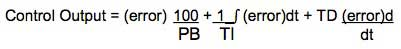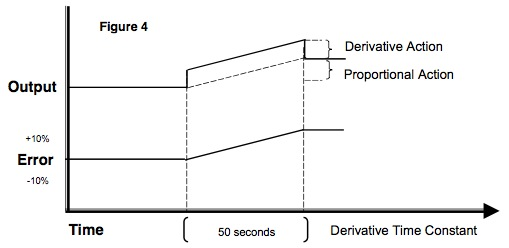The amount of derivative added to the control output is based in time units. Figure 4 below shows how derivative acts on the proportional band. The dashed line shows a proportional only control due to a process variable error from set point.

Using derivative (solid line), the control output jumps up, rises in a ramp and then falls back to proportional control action when the error becomes constant. In essence, it applies the "brakes" on the process error by quickly shifting the proportional band. Derivative has no effect on the output if the error is not changing. The derivative term should be approximately ¼ the integral time.

Derivative is typically not used in control loops with short dead/lag time, e. g., flow or pressure. This is an anticipatory action that will contribute to the inherent instability of these fast acting control loops.

### Related Products & Solutions

#### YS1700

The YS1700 Programmable Indicating Controller can be tailored for various applications by running a user program, and offers high reliability thanks to Yokogawa's proprietary technology, user friendliness, and expandability.

#### Single Loop Controllers

Single loop controllers receive temperature, flow rate, pressure, and other types of measurement readings from sensors and send corresponding instructions to control elements such as valves in order to maintain these values within a desired range.

×

### Have Questions?

Contact a Yokogawa Expert to learn how we can help you solve your challenges.

Top